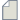Author Topic: Density difference  (Read 6262 times)

0 Members and 1 Guest are viewing this topic.ipsecog

• Regular ATK user
•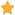• Posts: 35
• Country: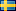• Reputation: 0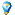Density difference
« on: December 3, 2008, 15:06 »
NanoLanguage doesn't allow you to subtract two ElectronDensity objects from each other (it does allow you to add them, however), which means it's not immediately obvious how to calculate e.g. the spin polarization density. By using some undocumented tricks, it is however possible to do it relatively easily anyway. Disclaimer: The scripts below use undocumented features, and may not work in future (and/or some old) releases of ATK. The steps involved are: 1) Extract the actual array data from the ElectronDensity objects. This is easy, just use the toArray() method on the object. 2) Calculate the difference. This is also easy, because the arrays (not the objects!) support the minus operation. 3) Store the difference. This is the tricky bit, but all we need to know is that the density data is stored in a private property called _ElectronDensity__electron_density_data. The second part of the trick is to use the Python module copy to create a new ElectronDensity object (since this class has no constructor exposed in NanoLanguage). Putting this together in a utility function, we obtain
Code
def calculateDensityDifference (d1,d2):

import copy

n1 = d1.toArray()
n2 = d2.toArray()
density = copy.copy(d1)
density._ElectronDensity__electron_density_data = (n1-n2)
return density
Calling this with two densities (d1 and d2), the function returns their difference in an ElectronDensity object, which you can put into a VNL file just as normal, and plot in Virtual NanoLab.ipsecog

• Regular ATK user
•• Posts: 35
• Country:• Reputation: 0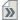Re: Density difference
« Reply #1 on: December 3, 2008, 15:08 »
Here is an example, using the function above! This is based on the famous Li-H2-Li example in the manual, and intends to calculate and plot the change in the self-consistent electron density at 0.7 V bias compared to the zero-bias case.
Code
from ATK.TwoProbe import *

def calculateDensityDifference (d1,d2):

import copy

n1 = d1.toArray()
n2 = d2.toArray()
density = copy.copy(d1)
density._ElectronDensity__electron_density_data = (n1-n2)
return density

scf1 = restoreSelfConsistentCalculation ('lih2li-bias-0.0.nc')
scf2 = restoreSelfConsistentCalculation ('lih2li-bias-0.7.nc')
density1 = calculateElectronDensity(scf1)   # 0.0 V bias
density2 = calculateElectronDensity(scf2)   # 0.7 V bias
diff_density = calculateDensityDifference(density1,density2)

f = VNLFile('lih2li.vnl')
The resulting plot from VNL is shown in the attached figure! (Coming as soon as I've calculated it!)ipsecog

• Regular ATK user
•• Posts: 35
• Country:• Reputation: 0Re: Density difference
« Reply #2 on: December 3, 2008, 15:11 »
Another example: How to calculate the spin polarization. This script performs a (simplified) self-consistent calculation of an oxygen (O2) molecule and afterwards computes and stores in the VNL file the spin polarization density (spin up density minus spin down density).
Code
from ATK.KohnSham import *

# Define the geometry for O2 molecule:
elements = [Oxygen, Oxygen]
coordinates =  [ (0.0,0.0,0.6) , (0.0,0.0,-0.6) ]*Ang
o2 = MoleculeConfiguration(elements, coordinates)

# Set the initial density with a maximum scaled spin:
initial_electron_density = electronDensityParameters(
initial_scaled_spin=(1,1)
)

# Define the calculation method:
dft_method=KohnShamMethod(
electron_density_parameters=initial_electron_density,
basis_set_parameters = basisSetParameters (type = SingleZeta)
)

import ATK
ATK.setCheckpointFilename("o2.nc")
ATK.setVerbosityLevel (level = 1)

# And execute the calculation:
scf=dft_method.apply(o2)

def calculateSpinDensityDifference (spin_density):

import copy

n_up = spin_density.toArray()
n_dn = spin_density.toArray()
new_density = copy.copy(spin_density)
new_density._ElectronDensity__electron_density_data = (n_up-n_dn)
return new_density

density = calculateElectronDensity(scf)
dens_diff = calculateSpinDensityDifference(density)

f = VNLFile('o2.vnl')